Computational & Technology Resources an online resource for computational,engineering & technology publications not logged in - login Civil-Comp ProceedingsISSN 1759-3433 CCP: 73PROCEEDINGS OF THE EIGHTH INTERNATIONAL CONFERENCE ON CIVIL AND STRUCTURAL ENGINEERING COMPUTING Edited by: B.H.V. Topping Paper 54Effects of Torsion on the Flexural Stiffness of the Rectangular Reinforced Concrete Sections M.J. Fadaee and M. BanihashemiDepartment of Civil Engineering, Shahid Bahonar University of Kerman, Iran doi:10.4203/ccp.73.54 Full Bibliographic Reference for this paper M.J. Fadaee, M. Banihashemi, "Effects of Torsion on the Flexural Stiffness of the Rectangular Reinforced Concrete Sections", in B.H.V. Topping, (Editor), "Proceedings of the Eighth International Conference on Civil and Structural Engineering Computing", Civil-Comp Press, Stirlingshire, UK, Paper 54, 2001. doi:10.4203/ccp.73.54 Keywords: bending moment, torsion, stiffness, reinforced concrete. Summary In recent years, much progress has been achieved in research on the behaviour of the reinforced concrete (RC) sections subjected to pure torsion. The proposed theories correspond experimental results very well, and hopefully, uniform codes for torsion can be available in near future, the same as what are now available for pure bending. There are many works in the literature investigating the behaviour of RC sections under combined actions (combination of axial force, bending moment and torsion) based upon truss theory. However, in different theories the angle of struts is assumed to have different values along the concrete member. By introducing the compression field theory and discovering the "softening phenomenon" the most important difficulties for finding the results more corresponding to the experimental results were dissolved. The modified compression field theory is known as "diagonal compression field theory" which is used for analysing the reinforced concrete sections subjected to combined loadings. In diagonal compression field theory, a RC section under combined actions is converted to a hollow thin-walled section. Any thin wall of the section is divided into several elements. For each element the concrete surface strain, the steel bar strain and the strut angle are different from the corresponding values for the other elements. So, three variables are considered for each element. If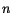is the total number of the section elements, there will be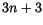non-linear equation to be solved in order to find the section response. Thus, as the total number of the elements increases, the number of the equations increases as well. In this paper, a progressive algorithm is presented for finding the response of the section under combined loadings. In the proposed method, it is proven that for finding the response of the section under combined loadings, there are always two independent variables and the other variables can be related to these two variables. In this method the procedure of finding the section response is done inversely. In the usual methods for solving the set of non-linear equations, the section actions are known while the bending curvature of the section,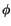, the torsion angle,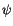, and the strain at section centeroid,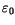, are the problem unknowns. In the proposed method,andare assumed to be known and the goal is finding the actions that produce such section response. In this method, contrary to the previous methods, when the total number of the elements increases, the number of the related variables increases only, and there are always two independent variables. Therefore, it is possible to increase the number of the elements in order to increase the accuracy of the solution without increasing the complexity of the solution. Adopting a constant value forand changingand using the proposed algorithm to find the actions that produce such response, a set of actions will be obtained. Adopting a new constant value forand changingagain, a new set of actions will be determined. At the end of the paper, the method is applied for an example section and the results are summarized in a table. Using the table the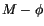curves for different values of torsion are plotted. The curves can be used in order to investigate the effect of torsion on the bending stiffness of the section. The proposed method gives the section response more accurately in comparison to the method based upon solving the set of non-linear equations. Moreover, in the proposed method there is no need to start from a point close to the true answer regarding the independent variables and, therefore, the answers are obtained much faster. References 1 Thomas T. C. Hsu, "Softened truss model theory for shear and torsion", ACI Structural Journal, 85(6), 624-635, 1988. 2 Thomas T. C. Hsu, "Shear flow zone in torsion of reinforced concrete", Journal of Structural Engineering, 116(11), 3206-3226, 1990. doi:10.1061/(ASCE)0733-9445(1990)116:11(3206) 3 Thomas T. C. Hsu, "Non-linear analysis of concrete membrane elements", ACI Structural Journal, 88(5), 552-561, 1991. 4 Thomas T. C. Hsu, "Non-linear analysis of concrete torsional members", ACI Structural Journal, 88(6), 674-682, 1991. 5 M. P. Collins and N.R. Khaldoun, "Simple model for predicting torsional strength of reinforced and pre-stressed concrete sections", ACI Structural Journal, 93(6), 658-666, 1996. 6 M.B. Leung and W.C. Schnobrich, "Reinforced concrete beams subjected to bending and torsion", Journal of Structural Engineering, 11(2), 307-320, 1986. doi:10.1061/(ASCE)0733-9445(1987)113:2(307) 7 M. Banihashemi, "A New Method for Finding the Response of Rectangular Reinforced Concrete Sections under Combined Bending and Torsion", M. Sc. Thesis, Shahid Bahonar University of Kerman, Kerman, Iran, 2001. purchase the full-text of this paper (price £20) Back to top ©Civil-Comp Limited 2023 - terms & conditions